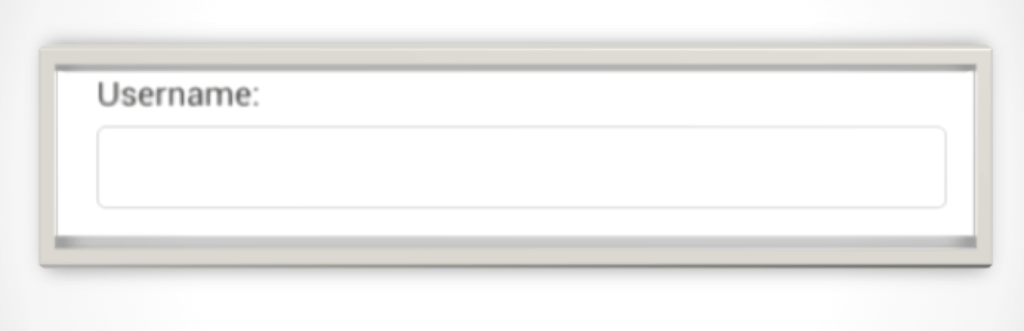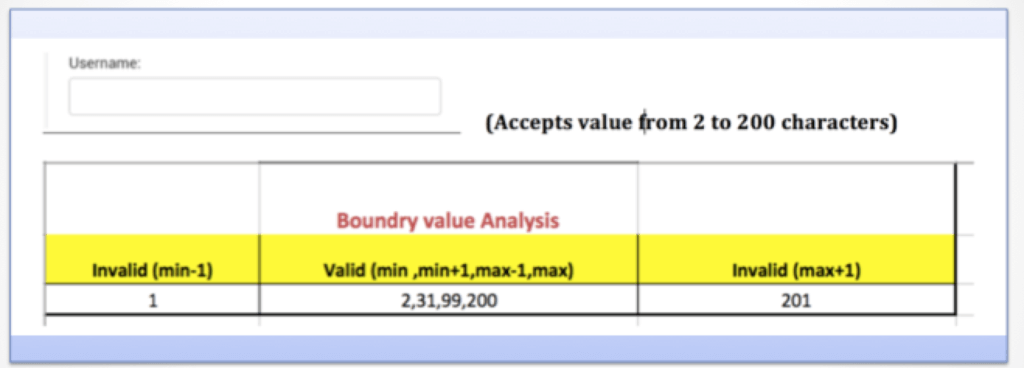Tutorials Hut

• # Equivalence partitioning and Boundry Value Analysis

Equivalence partitioning and Boundary value analysis are the techniques used to reduce the number of scenarios to test.

Equivalence Partitioning Method is also known as Equivalence class partitioning (ECP).

We are explaining the most important manual software testing techniques.

## Equivalence partitioning

•        It is also known as Equivalence Class Partitioning (ECP)
•        It is a specification-based or black box testing technique
•        The input data is divided into different equivalence data classes – which are generally termed as ‘Valid’ and ‘Invalid’.
•        Test only one condition from each partition or equivalence.
•        It reduces the number of test cases to a finite list of testable test cases covering maximum possibilities
•        Inputs are divided into groups that are expected to behave similar
•        Can be done for valid and invalid partitions
•        This can be applied at all levels/phases of testing
•        Partitions are also called Classes

Example:

Suppose In Login page A username field accepts character limit of 2 – 200 inclusive. Here, there would be three partitions: one valid partition and two invalid partitions.The valid partition: Between 2 & 200 characters. (Username field should handle all inputs with 2-200 characters)

The first invalid partition: less than 2 character any. Value for e.g. 1 (When less than 2 characters are entered, text field should reject the value)

The second invalid partition: > 200 (text field should reject all values which are greater than 200)

## Boundary Value analysis

• Boundary value analysis is a selection of test cases that takes boundary values as inputs. It is based on the assumption that errors are usually found on boundaries.
• It can be applied at all levels/phases of testing
• Boundaries are good place to look for defects
• Boundary Value Analysis is the next part of Equivalence Partitioning for designing test cases where test cases are selected at the edges of the equivalence classes.
• It is also a part of stress and negative testing.
• For each range, there are two boundaries, the lower boundary (start of the range) and the upper boundary (end of the range) and the boundaries are the beginning and end of each valid partition

Example:

A username on login page field accepts character limit of 2 – 200 inclusive.